##### Page tree
Go to start of banner

# Basic Data Types

## Constant values by the types

ValuePython Expression
Hexa decimal a10xa13.2e-12
• Horizontal Tab character
• Newline (ASCII Linefeed) character
• The character with hexadecimal value `a0`
• `'\t\n\xa0'`
• `"\t\n\xa0"`
• `'''\t\n\xa0'''`
• `"""\t\n\xa0"""`

## String

### String concatenation by join()

Write the code for a Python function expand(x) that takes a list of strings, concatenates them, and returns the resulting string repeated three times.

• Input: `['string1', 'string2']`
• Output: `'string1string2string1string2string1string2'`
```def expand(x):
return ''.join(x) * 3```

### Print string n times

```n = 2;
s ="Programming";

print(s * n); # ProgrammingProgramming```

# Basic Operators

## Python Arithmetic Operators

OperatorDescriptionExample
+ AdditionAdds values on either side of the operator.10 + 20 = 30
- SubtractionSubtracts right hand operand from left hand operand.10 – 20 = -10
* MultiplicationMultiplies values on either side of the operator10 * 20 = 200
/ DivisionDivides left hand operand by right hand operand20 / 10 = 2
% ModulusDivides left hand operand by right hand operand and returns remainder20 % 10 = 0
** ExponentPerforms exponential (power) calculation on operators10**20 =10 to the power 20
//Floor Division - The division of operands where the result is the quotient in which the digits after the decimal point are removed. But if one of the operands is negative, the result is floored, i.e., rounded away from zero (towards negative infinity) −

9//2 = 4 and 9.0//2.0 = 4.0

-11//3 = -4

-11.0//3 = -4.0

## Python Comparison Operators

Below example is based on the condition as a=10, b=20

OperatorDescriptionExample
==If the values of two operands are equal, then the condition becomes true.(a == b) is not true.
!=If values of two operands are not equal, then condition becomes true.(a != b) is true.
<>If values of two operands are not equal, then condition becomes true.(a <> b) is true. This is similar to != operator.
>If the value of left operand is greater than the value of right operand, then condition becomes true.(a > b) is not true.
<If the value of left operand is less than the value of right operand, then condition becomes true.(a < b) is true.
>=If the value of left operand is greater than or equal to the value of right operand, then condition becomes true.(a >= b) is not true.
<=If the value of left operand is less than or equal to the value of right operand, then condition becomes true.(a <= b) is true.

# Data Structures - List / Set / Tuple / Dictionary

## List

```list1 = ['physics', 'chemistry', 1997, 2000];
list2 = [1, 2, 3, 4, 5 ];
list3 = ["a", "b", "c", "d"]```

### Split string as list

```sentence = "the quick brown fox jumps over the lazy dog"
words = sentence.split()
print(words)```

### Filter positive numbers only - 1

```numbers = [34.6, -203.4, 44.9, 68.3, -12.2, 44.6, 12.7]
newlist = []
for number in numbers:
if number>0:
newlist.append(number)
print(newlist)```

### Filter positive numbers only - 2

```numbers = [34.6, -203.4, 44.9, 68.3, -12.2, 44.6, 12.7]
newlist = [int(x) for x in numbers if x > 0]
print(newlist)```

### Create word list from a sentence with no duplicate entries

set() removes all the duplicate entries in the array

```strings = "my name is Chun Kang and Chun is my name"
r = set(strings.split())
print(r)```

### Python List REPL sessions

```a = ['foo', 'bar', 'baz', 'qux', 'quux', 'corge']

print(a[:] is a)
print(max(a[2:4] + ['grault']))
print(a[-5:-3])
print(a[-6])
print(a[4::-2])```

Diagram for the list indices:

'foo''bar''baz''qux''quux''corge'
012345
-6-5-4-3-2-1

Result

```False
qux
['bar', 'baz']
foo
['quux', 'baz', 'foo']```

### Delete element in Python List

```a = [1, 2, 3, 4, 5]

# option 1 - delete element 3
del a

# option 2 - delete element 3
a.remove(3)

# option 3 - delete element 3
a[2:3] = []```

## Tuple

The tuples cannot be changed unlike lists and tuples use parentheses, whereas lists use square brackets.

```tup1 = ('physics', 'chemistry', 1997, 2000);
tup2 = (1, 2, 3, 4, 5 );
tup3 = "a", "b", "c", "d";```

### Ascending sort

```# set
pySet = {'e', 'a', 'u', 'o', 'i'}
print(sorted(pySet))

# dictionary
pyDict = {'e': 1, 'a': 2, 'u': 3, 'o': 4, 'i': 5}
print(sorted(pyDict))

# tuple
pyTuple = ('e', 'a', 'u', 'o', 'i')
print(sorted(pyTuple))```

### Descending sort

```# set
pySet = {'e', 'a', 'u', 'o', 'i'}
print(sorted(pySet, reverse=True))

# dictionary
pyDict = {'e': 1, 'a': 2, 'u': 3, 'o': 4, 'i': 5}
print(sorted(pyDict, reverse=True))

# tuple
pyTuple= ('e', 'a', 'u', 'o', 'i')
print(sorted(pyTuple, reverse=True))```

## Set

Unordered collections of unique elements

```Set(['Jane', 'Marvin', 'Janice', 'John', 'Jack'])
Set(['Janice', 'Jack', 'Sam'])
Set(['Jane', 'Zack', 'Jack'])
Set(['Jack', 'Sam', 'Jane', 'Marvin', 'Janice', 'John', 'Zack'])```

### Implement the unique_names by combining two lists

The returned list should have no duplicates and it must be sorted.

```def unique_names(names1, names2):
names1 += names2
return sorted( set(names1))

names1 = ["Ava", "Emma", "Olivia"]
names2 = ["Olivia", "Sophia", "Emma"]
print(unique_names(names1, names2)) # should print Ava, Emma, Olivia, Sophia```

### Find overlapped entries from two arrays

```a = set([ "Seoul", "Pusan", "Incheon", "Mokpo" ])
b = set([ "Seoul", "Incheon", "Suwon", "Daejeon", "Gwangjoo", "Taeku"])

print(a.intersection(b))
print(b.intersection(a))```

The result will be like below

Result

{'Seoul', 'Incheon'}

{'Seoul', 'Incheon'}

### Find different elements from two arrays based on "symmetric_difference" method

```a = set(["Jake", "John", "Eric"])
b = set(["John", "Jill"])

print(a.symmetric_difference(b))
print(b.symmetric_difference(a))```

The result will be like below

Result

{'Jake', 'Eric', 'Jill'}

{'Eric', 'Jake', 'Jill'}

### Find different elements from two arrays based on "difference" method

```a = set(["Jake", "John", "Eric"])
b = set(["John", "Jill"])

print(a.difference(b))
print(b.difference(a))```

The result will be like below

Result

{'Jake', 'Eric'}

{'Jill'}

### Find different elements from two arrays based on "union" method

```a = set(["Jake", "John", "Eric"])
b = set(["John", "Jill"])

print(a.union(b))```

The result will be like below

Result

{'John', 'Eric', 'Jake', 'Jill'}

### Print out a set containing all the participants from event A which did not attend event B

```a = ["Jake", "John", "Eric"]
b = ["John", "Jill"]

print(set(a).difference(set(b)))```

### Find sorted unique names in two list

```def unique_names(names1, names2):
return sorted(set(names1+names2))

names1 = ["Ava", "Emma", "Olivia"]
names2 = ["Olivia", "Sophia", "Emma"]
print(unique_names(names1, names2)) # should print Ava, Emma, Olivia, Sophia```

## Dictionary

Python dictionaries are similar to lists in that they are mutable and can be nested to any arbitrary depth (constrained only by available memory).

A dictionary can contain any type of Python object, including another dictionary. The keys in a given dictionary do not need to be the same type as one another, nor do the values.

Dictionary elements are accessed by key. Unlike with list indexing, the order of the items in a dictionary plays no role in how the items are accessed.

Even though dictionary access does not rely on item order, as of version 3.7 the Python language specification does guarantee that the order of items in a dictionary is maintained once the dictionary is created.

`dict = {'Name': 'Zara', 'Age': 7, 'Class': 'First'}`

### Get last name from full name by split()

The function can be easily implemented by string method

```actor = {"name": "John Cleese", "rank": "awesome"}

def get_last_name():
return actor["name"].split()

get_last_name()
print("All exceptions caught! Good job!")
print("The actor's last name is %s" % get_last_name())```

### Accessing dictionary values

```x = [
'a',
'b',
{
'foo': 1,
'bar':
{
'x' : 10,
'y' : 20,
'z' : 30
},
'baz': 3
},
'c',
'd'
]

print(x['bar']['z'])```

Result

`30`

### Delete a dictionary element

Deleting a dictionary element by statement

`del d['foo']`

Deleting a dictionary element by method

`d.pop('foo')`

### Copying a dictionary

Method 1)

`d2 = dict(d1)`

Method 2)

`d2 = dict(d1.items())`

Method 3)

```d2 = {}
d2.update(d1)```

## Hashmap

Python kindly provides a built-in type hashmap named as dictionary. You can easily hash like below:

```myHashmap = {}

# hash by name
myHashmap['Chun'] = 1234
myHashmap['John'] = 2342

# print hash value
print( myHashmap['Chun'])```

# Generator

## Random number generation

```import random

def lottery():
# returns 6 numbers between 1 and 40
for i in range(6):
yield random.randint(1, 40)

# returns a 7th number between 1 and 15
yield random.randint(1,15)

for random_number in lottery():
print("And the next number is... %d!" %(random_number))```

```a = 1
b = 2
a, b = b, a
print(a,b)```

## Fibonacci series generator

The first two numbers of the series is always equal to 1, and each consecutive number returned is the sum of the last two numbers - the below code uses only two variables to get the result.

```def fib():
a, b = 1, 1
while 1:
yield a
a, b = b, a + b

# testing code
import types
if type(fib()) == types.GeneratorType:
print("Good, The fib function is a generator.")

counter = 0
for n in fib():
print(n)
counter += 1
if counter == 10:
break```

# Function Arguments(Parameters)

## Multiple Function Argument recognition - the list of "therest" parameters

```def foo(first, second, third, *therest):
print("First: %s" %(first))
print("Second: %s" %(second))
print("Third: %s" %(third))
print("And all the rest... %s" %(list(therest)))

foo(1,2,3,4,5)```

## Multiple Function Argument by keyword

```def bar(first, second, third, **options):
if options.get("action") == "sum":
print("The sum is: %d" %(first + second + third))

if options.get("number") == "first":
return first

result = bar(1, 2, 3, action = "sum", number = "first")
print("Result: %d" %(result))```

# Regular Expression

## RegEx(Regular Expressions) to search "[on]" or "[off]" on the string

```import re

pattern = re.compile(r"\[(on|off)\]") # Slight optimization
print(re.search(pattern, "Mono: Playback 65 [75%] [-16.50dB] [on]"))```

## RegEx(Regular Expression) to check email address

```import re

def test_email(your_pattern):
pattern = re.compile(your_pattern)
emails = ["john@example.com", "python-list@python.org", "wha.t.`1an?ug{}ly@email.com"]
for email in emails:
if not re.match(pattern, email):
print("You failed to match %s" % (email))
elif not your_pattern:
print("Forgot to enter a pattern!")
else:
print("Pass")

pattern = r"[a-z0-9]+@[a-z0-9]+\.[a-z0-9]+"
test_email(pattern)```

# Exception Handling

## try/except block

```def do_stuff_with_number(n):
print(n)

def catch_this():
the_list = (1, 2, 3, 4, 5)

for i in range(20):
try:
do_stuff_with_number(the_list[i])
except IndexError: # Raised when accessing a non-existing index of a list
do_stuff_with_number('out of bound - %d' % i)

catch_this()
```

# Numpy

## Convert arrays to Numpy arrays

```# Create 2 new lists height and weight
height = [1.87,  1.87, 1.82, 1.91, 1.90, 1.85]
weight = [81.65, 97.52, 95.25, 92.98, 86.18, 88.45]

# Import the numpy package as np
import numpy as np

# Create 2 numpy arrays from height and weight
np_height = np.array(height)
np_weight = np.array(weight)

print(type(np_height))

# Calculate bmi
bmi = np_weight / np_height ** 2

# Print the result
print(bmi)

# For a boolean response
print(bmi > 23)

# Print only those observations above 23
print(bmi[bmi > 23])```

Result

```<class 'numpy.ndarray'>
[ 23.34925219  27.88755755  28.75558507  25.48723993  23.87257618
25.84368152]
[ True  True  True  True  True  True]
[ 23.34925219  27.88755755  28.75558507  25.48723993  23.87257618
25.84368152]```

## Convert all of the weights from kilograms to pounds based in NumPy

```weight_kg = [81.65, 97.52, 95.25, 92.98, 86.18, 88.45]

import numpy as np

# Create a numpy array np_weight_kg from weight_kg
np_weight_kg = np.array(weight_kg)

# Create np_weight_lbs from np_weight_kg
np_weight_lbs = np_weight_kg * 2.2

# Print out np_weight_lbs
print(np_weight_lbs)```

Result

`    [ 179.63   214.544  209.55   204.556  189.596  194.59 ]`

# Pandas DataFrame / CSV / Join / Merge

## Create a Pandas DataFrame based on array

```dict = {"country": ["Brazil", "Russia", "India", "China", "South Africa"],
"capital": ["Brasilia", "Moscow", "New Dehli", "Beijing", "Pretoria"],
"area": [8.516, 17.10, 3.286, 9.597, 1.221],
"population": [200.4, 143.5, 1252, 1357, 52.98] }

import pandas as pd
brics = pd.DataFrame(dict)
print(brics)```

## Adding index to a Pandas DataFrame

```# Set the index for brics
brics.index = ["BR", "RU", "IN", "CH", "SA"]

# Print out brics with new index values
print(brics)```

## Reading CSV by Pandas DataFrame

```# Import pandas as pd
import pandas as pd

# Import the cars.csv data: cars

# Print out cars
print(cars)```

## CSV

### Reading a CSV file by Pandas DataFrame with 1st column as index

```# Import pandas and cars.csv
import pandas as pd
cars = pd.read_csv('cars.csv', index_col = 0)

# Print out country column as Pandas Series
print(cars['cars_per_cap'])

# Print out country column as Pandas DataFrame
print(cars[['cars_per_cap']])

# Print out DataFrame with country and drives_right columns
print(cars[['cars_per_cap', 'country']])```

### Save a Pandas DaraFrame by CSV format

```dict = {"country": ["Brazil", "Russia", "India", "China", "South Africa"],
"capital": ["Brasilia", "Moscow", "New Dehli", "Beijing", "Pretoria"],
"area": [8.516, 17.10, 3.286, 9.597, 1.221],
"population": [200.4, 143.5, 1252, 1357, 52.98] }

import pandas as pd
brics = pd.DataFrame(dict)

brics.to_csv('example.csv')```

### Save a Pandas DaraFrame by CSV format with header and no index

```from pandas import DataFrame

Cars = {'Brand': ['Honda Civic','Toyota Corolla','Ford Focus','Audi A4'],
'Price': [22000,25000,27000,35000]
}

df = DataFrame(Cars, columns= ['Brand', 'Price'])

export_csv = df.to_csv (r'C:\Users\Ron\Desktop\export_dataframe.csv', index = None, header=True) #Don't forget to add '.csv' at the end of the path

print (df)```

### Print partial rows (observations) from a Pandas DataFrame

```# Import cars data
import pandas as pd
cars = pd.read_csv('cars.csv', index_col = 0)

# Print out first 4 observations
print(cars[0:4])

# Print out fifth, sixth, and seventh observation
print(cars[4:6])```

## Data access by loc and iloc in Pandas DaraFrame - Select colums by index or name

loc is label-based, and iloc is integer index based

```# Import cars data
import pandas as pd
cars = pd.read_csv('cars.csv', index_col = 0)

# Print out observation for Japan
print(cars.iloc)

# Print out observations for Australia and Egypt
print(cars.loc[['AUS', 'EG']])```

## Sort

### Sort a Pandas DataFrame in an ascending order

`df.sort_values(by=['Brand'], inplace=True)`
```# sort - ascending order
from pandas import DataFrame

Cars = {'Brand': ['Honda Civic','Toyota Corolla','Ford Focus','Audi A4'],
'Price': [22000,25000,27000,35000],
'Year': [2015,2013,2018,2018]
}

df = DataFrame(Cars, columns= ['Brand', 'Price','Year'])

# sort Brand - ascending order
df.sort_values(by=['Brand'], inplace=True)

print (df)```

### Sort a Pandas DataFrame in a descending order

`df.sort_values(by=['Brand'], inplace=True, ascending=False)`
```# sort - descending order
from pandas import DataFrame

Cars = {'Brand': ['Honda Civic','Toyota Corolla','Ford Focus','Audi A4'],
'Price': [22000,25000,27000,35000],
'Year': [2015,2013,2018,2018]
}

df = DataFrame(Cars, columns= ['Brand', 'Price','Year'])

# sort Brand - descending order
df.sort_values(by=['Brand'], inplace=True, ascending=False)

print (df)```

### Sort a Pandas DataFrame by multiple columns

`df.sort_values(by=['First Column','Second Column',...], inplace=True)`
```# sort by multiple columns
from pandas import DataFrame

Cars = {'Brand': ['Honda Civic','Toyota Corolla','Ford Focus','Audi A4'],
'Price': [22000,25000,27000,35000],
'Year': [2015,2013,2018,2018]
}

df = DataFrame(Cars, columns= ['Brand', 'Price','Year'])

# sort by multiple columns: Year and Price
df.sort_values(by=['Year','Price'], inplace=True)

print (df)```

## Join and merge Pandas DataFrames

```import pandas as pd
from IPython.display import display
from IPython.display import Image

raw_data = {
'subject_id': ['1', '2', '3', '4', '5'],
'first_name': ['Alex', 'Amy', 'Allen', 'Alice', 'Ayoung'],
'last_name': ['Anderson', 'Ackerman', 'Ali', 'Aoni', 'Atiches']}
df_a = pd.DataFrame(raw_data, columns = ['subject_id', 'first_name', 'last_name'])

raw_data = {
'subject_id': ['4', '5', '6', '7', '8'],
'first_name': ['Billy', 'Brian', 'Bran', 'Bryce', 'Betty'],
'last_name': ['Bonder', 'Black', 'Balwner', 'Brice', 'Btisan']}
df_b = pd.DataFrame(raw_data, columns = ['subject_id', 'first_name', 'last_name'])

raw_data = {
'subject_id': ['1', '2', '3', '4', '5', '7', '8', '9', '10', '11'],
'test_id': [51, 15, 15, 61, 16, 14, 15, 1, 61, 16]}
df_n = pd.DataFrame(raw_data, columns = ['subject_id','test_id'])

# Join the two dataframes along rows
df_new = pd.concat([df_a, df_b])

# Join the two dataframes along columns
pd.concat([df_a, df_b], axis=1)

# Merge two dataframes along the subject_id value
pd.merge(df_new, df_n, on='subject_id')

# Merge two dataframes with both the left and right dataframes using the subject_id key
pd.merge(df_new, df_n, left_on='subject_id', right_on='subject_id')

# Merge with outer join
pd.merge(df_a, df_b, on='subject_id', how='outer')

# Merge with inner join
pd.merge(df_a, df_b, on='subject_id', how='inner')

# Merge with right join
pd.merge(df_a, df_b, on='subject_id', how='right')

# Merge with left join
pd.merge(df_a, df_b, on='subject_id', how='left')

# Merge while adding a suffix to duplicate column names
pd.merge(df_a, df_b, on='subject_id', how='left', suffixes=('_left', '_right'))

# Merge based on indexes
pd.merge(df_a, df_b, right_index=True, left_index=True)```

## Get the maximum value of column in Pandas DataFrame

```import pandas as pd

# Create a DataFrame
d = {
'Name':['Alisa','Bobby','jodha','jack','raghu','Cathrine',
'Alisa','Bobby','kumar','Alisa','Alex','Cathrine'],
'Age':[26,24,23,22,23,24,26,24,22,23,24,24],

'Score':[85,63,55,74,31,77,85,63,42,62,89,77]
}

df = pd.DataFrame(d,columns=['Name','Age','Score'])

# get the maximum values of all the column in dataframe - it will be raghu, 26, 89, object
df.max()

# get the maximum value of the column 'Age' - it will be 26
df['Age'].max()

# get the maximum value of the column 'Name' - it will be raghu
df['Name'].max()```

## Get the minimum value of column in Pandas DataFrame

```import pandas as pd

# Create a DataFrame
d = {
'Name':['Alisa','Bobby','jodha','jack','raghu','Cathrine',
'Alisa','Bobby','kumar','Alisa','Alex','Cathrine'],
'Age':[26,24,23,22,23,24,26,24,22,23,24,24],

'Score':[85,63,55,74,31,77,85,63,42,62,89,77]
}

df = pd.DataFrame(d,columns=['Name','Age','Score'])

# get the minimum values of all the column in dataframe - it will display Alex, 22, 31, object
df.min()

# get the minimum value of the column 'Age' - it will be 22
df['Age'].min()

# get the minimum value of the column 'Name' - it will be Alex
df['Name'].min()```

## Select row with maximum and minimum value in Pandas DataFrame

```import pandas as pd

# Create a DataFrame
d = {
'Name':['Alisa','Bobby','jodha','jack','raghu','Cathrine',
'Alisa','Bobby','kumar','Alisa','Alex','Cathrine'],
'Age':[26,24,23,22,23,24,26,24,22,23,24,24],

'Score':[85,63,55,74,31,77,85,63,42,62,89,77]}

df = pd.DataFrame(d,columns=['Name','Age','Score'])

# get the row of max value
df.loc[df['Score'].idxmax()]

# get the row of minimum value
df.loc[df['Score'].idxmin()]```

## Get the unique values (rows) of a Pandas Dataframe

```import pandas as pd

# Create a DataFrame
d = {
'Name':['Alisa','Bobby','jodha','jack','raghu','Cathrine',
'Alisa','Bobby','kumar','Alisa','Alex','Cathrine'],
'Age':[26,24,23,22,23,24,26,24,22,23,24,24]
}

df = pd.DataFrame(d,columns=['Name','Age'])

# get the unique values (rows)
print df.drop_duplicates()

# get the unique values (rows) by retaining last row
print df.drop_duplicates(keep='last')```

## Get the list of column headers or column name in a Pandas DataFrame

```import pandas as pd

# Create a DataFrame
d = {
'Name':['Alisa','Bobby','jodha','jack','raghu','Cathrine',
'Alisa','Bobby','kumar','Alisa','Alex','Cathrine'],
'Age':[26,24,23,22,23,24,26,24,22,23,24,24],

'Score':[85,63,55,74,31,77,85,63,42,62,89,77]}

df = pd.DataFrame(d,columns=['Name','Age','Score'])

# method 1: get list of column name
list(df.columns.values)

# method 2: get list of column name
list(df)```

## Delete or Drop the duplicate row of a Pandas DataFrame

```import pandas as pd

# Create a DataFrame
d = {
'Name':['Alisa','Bobby','jodha','jack','raghu','Cathrine',
'Alisa','Bobby','kumar','Alisa','Alex','Cathrine'],
'Age':[26,24,23,22,23,24,26,24,22,23,24,24],

'Score':[85,63,55,74,31,77,85,63,42,62,89,77]}

df = pd.DataFrame(d,columns=['Name','Age','Score'])

# drop duplicate rows
df.drop_duplicates()

# drop duplicate rows by retaining last occurrence
df.drop_duplicates(keep='last')

# drop duplicate by a column name
df.drop_duplicates(['Name'], keep='last')```

## Drop or delete the row in Pandas DataFrame with conditions

```import pandas as pd

# Create a DataFrame
d = {
'Name':['Alisa','Bobby','jodha','jack','raghu','Cathrine',
'Alisa','Bobby','kumar','Alisa','Alex','Cathrine'],
'Age':[26,24,23,22,23,24,26,24,22,23,24,24],

'Score':[85,63,55,74,31,77,85,63,42,62,89,77]}

df = pd.DataFrame(d,columns=['Name','Age','Score'])

# Drop an observation or row
df.drop([1,2])

# Drop a row by condition
df[df.Name != 'Alisa']

# Drop a row by index
df.drop(df.index)

# Drop bottom 3 rows
df[:-3]```

## Reshape wide to long in Pandas DataFrame with melt() function

```import pandas as pd

# Create a DataFrame
d = {
'countries':['A','B','C'],
'population_in_million':[100,200,120],
'gdp_percapita':[2000,7000,15000]
}

df = pd.DataFrame(d,columns=['countries','population_in_million','gdp_percapita'])

# shape from wide to long with melt function in pandas
df2=pd.melt(df,id_vars=['countries'],var_name='metrics', value_name='values')```

## Reshape long to wide in Pandas DataFrame with pivot function

```import pandas as pd

# Create a DataFrame
d = {
'countries':['A','B','C','A','B','C'],
'metrics':['population_in_million','population_in_million','population_in_million',
'gdp_percapita','gdp_percapita','gdp_percapita'],
'values':[100,200,120,2000,7000,15000]
}

df = pd.DataFrame(d,columns=['countries','metrics','values'])

# reshape from long to wide in pandas python
df2=df.pivot(index='countries', columns='metrics', values='values')

```

## Reshape using Stack() and unstack() function in Pandas DataFrame

```import pandas as pd

d=([[12,45,67,56],[78,89,45,67],[45,67,89,90],[67,44,56,55]])

df = pd.DataFrame(d,
index=['Alisa','Bobby','Cathrine','Jack'],

# stack the dataframe
stacked_df=df.stack()

# unstack the dataframe
unstacked_df = stacked_df.unstack()

# stack the dataframe of column at level 0
stacked_df_lvl=df.stack(level=0)

# unstack the dataframe
unstacked_df1 = stacked_df_lvl.unstack()```

# MatPlotLib

## Line chart by city and population by MatPlotLib

```from matplotlib import pyplot as plt

plt.plot(["Seoul","Paris","Seattle"], [30,25,55])
plt.xlabel('City')
plt.ylabel('Response')
plt.title('Experiment Result')
plt.show()```

Result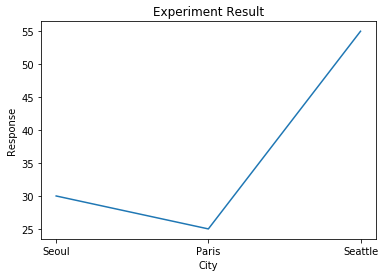## Bar chart by city and population by MatPlotLib

```from matplotlib import pyplot as plt

plt.bar(["Seoul","Paris","Seattle"], [30,25,55])
plt.xlabel('City')
plt.ylabel('Response')
plt.title('Experiment Result')
plt.show()```

Result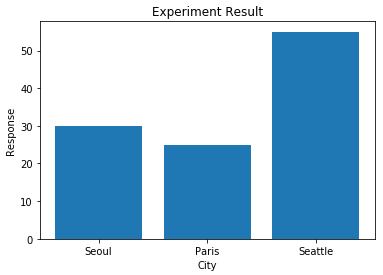## Spot graph by age and score by MapPlotLib

Below code shows a graph displaying multiple spots by age and score stored in a pandas data frame.

```import pandas as pd
from matplotlib import pyplot as plt

# Create a DataFrame
d = {
'Name':['Alisa','Bobby','jodha','jack','raghu','Cathrine',
'Alisa','Bobby','kumar','Alisa','Alex','Cathrine'],
'Age':[26,24,23,22,23,24,26,24,22,23,24,24],

'Score':[85,63,55,74,31,77,85,63,42,62,89,77]
}

df = pd.DataFrame(d,columns=['Name','Age','Score'])

plt.plot(df['Age'], df['Score'], 'ro')
plt.xlabel('Age')
plt.ylabel('Score')
plt.title('Experiment Result')
plt.show()```

Result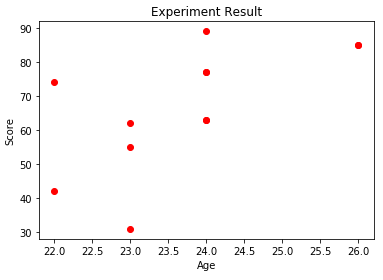## Draw Samsung's stock price by MatPlotLib

```import matplotlib
matplotlib.use('Agg')

import pandas as pd

import matplotlib
matplotlib.use('Agg')

from matplotlib import pyplot as plt

import os

# samsung.set_index( 'Date', inplace=True)

print("<pre>")

print(samsung)

print("\n")

max_date = samsung['Date'].max()
print('Last date of record stored %s' % max_date)

print("</pre>")

if not os.path.exists('./samsung_stock_price.png'):
plt.plot(samsung['Date'], samsung['Close'])
plt.xlabel('Date')
plt.ylabel('Close')
plt.title('Experiment Result')
plt.savefig('samsung_stock_price.png')

print("<img src=samsung_stock_price.png>")

```

Result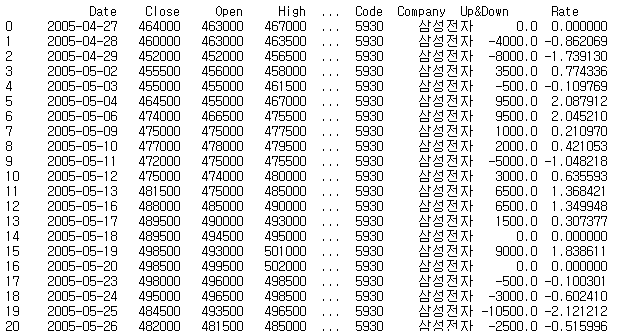...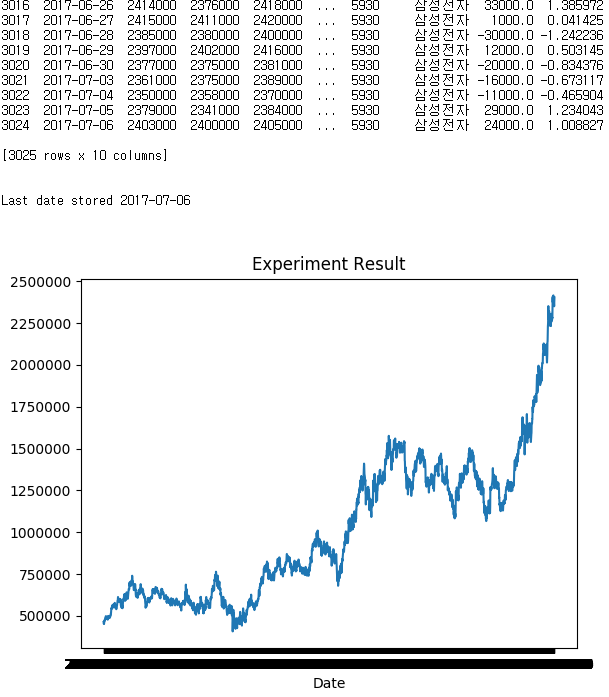# OS

## Check if file exists or not

```import os

if not os.path.exists('test.png'):
# do something if 'test.png' does not exist
pass

```

# Algorithms

## Check all unique or not

```def all_unique(lst):
return len(lst) == len(set(lst))

x = [1,1,2,2,3,2,3,4,5,6]
y = [1,2,3,4,5]
all_unique(x) # False
all_unique(y) # True```

## Palindrome number - Determine whether an integer is a palindrome

```def is_palindrome(word):
j = len(word)-1
i =0
while i<j and word[i].lower()==word[j].lower():
i+=1
j-=1

return (i>=j)

print(is_palindrome('Deleveled'))```

## Two sum -  return indices of the two numbers such that they add up to a specific target

Given nums = [2, 7, 11, 15], target = 9,

Because nums + nums = 2 + 7 = 9,
return [0, 1].

```def twoSum(self, nums, target):
seen = {}
for i, v in enumerate(nums):
remaining = target - v
if remaining in seen:
return [seen[remaining], i]
seen[v] = i
return []```

## Reverse integer - Given a 32-bit signed integer, reverse digits of an integer.

Input: 123 → Output: 321
Input: -123 → Output: -321
Input: 120 → Output: 21

```class Solution(object):
def reverse(self, x):
if x >= 2**31-1 or x <= -2**31:
return 0
else:
strg = str(x)

if x >= 0 :
revst = strg[::-1]
else:
temp = strg[1:]
temp2 = temp[::-1]
revst = "-" + temp2

if int(revst) >= 2**31-1 or int(revst) <= -2**31:
return 0
else:
return int(revst)```

## Merge two sorted linked lists and return it as a new list.

Input: 1->2->4, 1->3->4
Output: 1->1->2->3->4->4

```class Solution:
def mergeTwoLists(self, l1, l2):

result = ListNode(0) # The new list we are going to eventually return
head = result # keep a pointer to the head so we can return head.next in the end
while(l1 != None and l2 != None): # This check is important in the case where one list is shorter than the other
if l1.val < l2.val: # Add l1's value as a new node to result if its less than l2's
result.next = ListNode(l1.val)
l1 = l1.next
result = result.next
elif l2.val < l1.val: # Add l2's value as a new node to result if its less than l1's
result.next = ListNode(l2.val)
l2 = l2.next
result = result.next
else: # In this case, the values must be equal so add both to result and move the linked lists forward
result.next = ListNode(l1.val)
result = result.next
result.next = ListNode(l2.val)
result = result.next
l1 = l1.next
l2 = l2.next

if l1 == None and l2 != None: # If l2 is longer than l1, add all of the remaining values of l2 to result
while(l2 != None):
result.next = ListNode(l2.val)
result = result.next
l2 = l2.next
elif l2 == None and l1 != None: # if l1 is longer than l2, add all of the remaining values of l1 to result
while(l1 != None):
result.next = ListNode(l1.val)
result = result.next
l1 = l1.next

return head.next # return the result```

## Remove Duplicates from Sorted Array

```class Solution(object):
def removeDuplicates(self, nums):
if not nums:
return 0

i = 1
bound = len(nums)
prev = nums

while i < bound:
if prev == nums[i]:
nums.pop(i)
bound = len(nums)
else:
prev = nums[i]
i += 1

return len(nums)```

## Longest common prefix

Input: ["flower", "flow", "flight" ] → Output: "fl"

Input: ["dog", "racecar", "car"] → Output: ""

```class Solution:
def longestCommonPrefix(self, strs: List[str]) -> str:
if not strs:
return ""

result=""
cnt=len(strs)
for i in range(len(strs)):
j=1
while j<cnt and i<len(strs[j]) and strs[j][i]==strs[i]: j+=1

if j==cnt:
result += strs[i]
else:
break;

return result;```

## Longest Palindromic Substring

Input: "cbd" → Output: "bb"

```class Solution:
def longestPalindrome(self, s):
"""
:type s: str
:rtype: str
"""
# Return if string is empty
if not s: return s

res = ""
for i in range(len(s)):
j = i + 1
# While j is less than length of string
# AND res is *not* longer than substring s[i:]
while j <= len(s) and len(res) <= len(s[i:]):
# If substring s[i:j] is a palindrome
# AND substring is longer than res
if s[i:j] == s[i:j][::-1] and len(s[i:j]) > len(res):
res = s[i:j]
j += 1

return res```

## Same Tree

```# Definition for a binary tree node.
# class TreeNode:
#     def __init__(self, x):
#         self.val = x
#         self.left = None
#         self.right = None

class Solution:
def isSameTree(self, p: TreeNode, q: TreeNode) -> bool:
if p==None and q!=None: return False
if p!=None and q==None: return False
if p==None and q==None: return True

if p.val==q.val:
return self.isSameTree(p.left, q.left) and self.isSameTree(p.right, q.right)```

## Merge Sorted Array

Given two sorted integer arrays nums1 and nums2, merge nums2 into nums1 as one sorted array.

nums1 = [1,2,3,0,0,0], m = 3
nums2 = [2,5,6], n = 3

Output: [1,2,2,3,5,6]

```class Solution:
def merge(self, nums1: List[int], m: int, nums2: List[int], n: int) -> None:
"""
Do not return anything, modify nums1 in-place instead.
"""
p = m+n-1
m -= 1
n -= 1
while p>=0:
if n<0:
break;
elif m<0:
nums1[p] = nums2[n]
n-=1
elif nums1[m]<nums2[n]:
nums1[p] = nums2[n]
n-=1
else:
nums1[p] = nums1[m]
m-=1
p-=1```

## Minimum Depth of Binary Tree

Given binary tree [3,9,20,null,null,15,7],

3
/ \
9 20
/ \
15 7
return its minimum depth = 2.

```# Definition for a binary tree node.
# class TreeNode:
#     def __init__(self, x):
#         self.val = x
#         self.left = None
#         self.right = None

class Solution:
def minDepth(self, root: TreeNode) -> int:
if root==None:
return 0

if root.left==None and root.right==None: return 1
elif root.left!=None and root.right!=None:
return min( self.minDepth(root.left), self.minDepth(root.right)) + 1
elif root.left==None:
return self.minDepth(root.right) + 1
else:
return self.minDepth(root.left) + 1

```

## Reorder List

Given a singly linked list L: L0→L1→…→Ln-1→Ln,
reorder it to: L0→Ln→L1→Ln-1→L2→Ln-2→…

ex1) Given 1->2->3->4, reorder it to 1->4->2->3.
ex2) Given 1->2->3->4->5, reorder it to 1->5->2->4->3.

```# Definition for singly-linked list.
# class ListNode:
#     def __init__(self, x):
#         self.val = x
#         self.next = None

class Solution:
def reorderList(self, head: ListNode) -> None:
"""
"""

# collect value serially
v=[]
while p!=None:
v.append(p.val)
p=p.next

# reorder based on the given logic
i=0
lcnt=len(v)
j=lcnt-1
while p!=None:
p.val = v[i]
i += 1
p=p.next

if p!=None:
p.val = v[j]
j-=1
p = p.next
```

## Compact - Remove Falsy values (None, False, "")

```def compact(lst):
return list(filter(None, lst))

compact([0, 1, False, 2, '', 3, 'a', 's', 34]) # [ 1, 2, 3, 'a', 's', 34 ]```

# Python Simple Web Server

simple_http_server.py
```import SimpleHTTPServer
import SocketServer

PORT = 8888

Handler = SimpleHTTPServer.SimpleHTTPRequestHandler

httpd = SocketServer.TCPServer(("", PORT), Handler)

print "serving at port", PORT
httpd.serve_forever()```

You can run above as

`python -m simple_http_server.py`